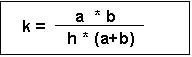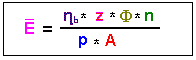The lumen method serves to provide approximate calculations for planning indoor lighting systems. The method is used to determine the number of luminaires and lamps required to achieve a given Illuminance. This first step is usually followed by more accurate calculations to determine whether the requirements of Uniformity and other conditions for a good interior lighting system have been met (cf. Ten Guidelines for Right Light).

Comparable methods for calculating mean illuminance have been developed and published by other national and international bodies. All these methods are based on the same assumptions as far as calculations of the indirect portion of light are concerned, but they differ in the methods used to calculate direct utilised flux and in their tabular form of presentation of calculated efficiency.

The results depend on room geometry and reflectance, the Luminous Intensity Distribution of the luminaires and their configuration in the room. The accuracy of the luminaire data used is also critical. Apart from that, the lumen methodis based on a number of assumptions made to ensure that the method is easy to use. The more actual conditions deviate from these assumptions in the individual case, the less accurate the calculations.

The basic assumptions underlying the lumen method :

• rectangular room,
• ratio of length to width = 1.6:1, with a maximum of 4:1,
• completely empty room,
• uniform reflectance and completely diffuse reflection properties of the perimeter surfaces,
• uniform distribution of luminous flux over all areas,
• regular luminaire configuration throughout the room,
• in the case of fluorescent lamps, luminaire axis = room axis.

Procedure :

1. Determining the utilisation factor from the table of photometric data :

• Establish reflectance values for ceiling, walls and floor.
• Calculate room index k using the formula :a length of room [in m], b width of room [in m], h height above the working plane.
• Read off the utilisation factor ηB from the table of photometric data supplied with the luminaires (listed as %, i.e. 68% = 0.68).

2. Calculating the number of luminaires needed to achieve a given mean illuminance at the work plane :

• Derive Mean Illuminance En from the requirements to be met for the planned activity or type of interior (DIN 5035 Part 2).
• Select a luminaire.
• Calculate floor space A = length of room x width [in m²].
• Let the utilisation factor be ηB (from section 1).
• Let the number of lamps per luminaire be z.
• Let the rated luminous flux of a lamp be Φ [in lm].
• Determine the Design Factor p to take account of dirt accumulation in the room and the luminaires plus lamp ageing. The factor is usually 1.25, but this figure can be increased to take account of dirty or dusty environments.
• Calculate the number n of luminaires required.• Round off the value for n to obtain a whole number of luminaires and a logical luminaire configuration for the interior, e.g. 10.8 => 12 (for 3 x 4 luminaires).

3. Calculating mean illuminance E available at the work plane in the long term with the planned lighting system :

• Let the utilisation factor be ηB.
• Let the planned number of luminaires for the room be n.
• Let the number of lamps per luminaire be z.
• Let the rated luminous flux of a lamp be Φ.
• Let the design factor be p.
• Calculate floor space A.
• Calculate available mean illuminance E.Interior lighting systems often provide levels of illumination that deviate slightly from given or available levels of illuminance without causing any physiological problems. If a higher degree of accuracy is considered desirable in planning a lighting system, however, it should be borne in mind that the various national standards, recommendations and guidelines usually lay down minimum values for illuminance which must be met. On the other hand, exceeding such minimum values to a significant degree in the installation of a lighting system causes higher costs which are almost proportional to the excess illuminance.

For practical reasons (tolerances in the photometric data of lamps and luminaires, deviations from the operating conditions assumed for the calculations, e.g. temperature, mains voltage etc., or from the reflectance values for the room perimeters, plus measuring inaccuracies), deviations in the range of ±10% constitute a good result in terms of agreement between the calculations and the actual values measured for the finished system. Better results can only be achieved by employing more complicated procedures - and thus incurring unduly high costs - to quantify the individual parameters within the system.

A greater degree of accuracy, especially in terms of more detail with regard to the distribution of illuminance at the work plane and room surfaces, plus realistic visualisation of the expected lighting effects on the interior, are available with the DIALux computer program.